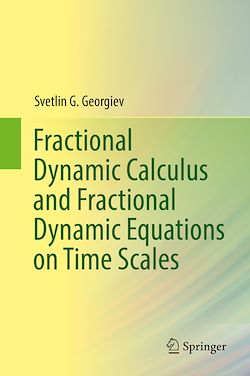# Fractional Dynamic Calculus and Fractional Dynamic Equations on Time Scales

-English
360 Pages

Description

Pedagogically organized, this monograph introduces fractional calculus and fractional dynamic equations on time scales in relation to mathematical physics applications and problems. Beginning with the definitions of forward and backward jump operators, the book builds from Stefan Hilger’s basic theories on time scales and examines recent developments within the field of fractional calculus and fractional equations. Useful tools are provided for solving differential and integral equations as well as various problems involving special functions of mathematical physics and their extensions and generalizations in one and more variables. Much discussion is devoted to Riemann-Liouville fractional dynamic equations and Caputo fractional dynamic equations.
Intended for use in the field and designed for students without an extensive mathematical background, this book is suitable for graduate courses and researchers looking for an introduction to fractional dynamic calculus and equations on time scales.

Subjects

##### Calculus

Informations